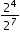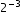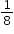# MA.8.NSO.1.3

Extend previous understanding of the Laws of Exponents to include integer exponents. Apply the Laws of Exponents to evaluate numerical expressions and generate equivalent numerical expressions, limited to integer exponents and rational number bases, with procedural fluency.

### Examples

The expressionis equivalent towhich is equivalent to.

### Clarifications

Clarification 1: Refer to the K-12 Formulas (Appendix E) for the Laws of Exponents.
General Information
Subject Area: Mathematics (B.E.S.T.)
Strand: Number Sense and Operations
Date Adopted or Revised: 08/20
Status: State Board Approved

## Related Courses

This benchmark is part of these courses.
1205050: M/J Accelerated Mathematics Grade 7 (Specifically in versions: 2014 - 2015, 2015 - 2020, 2020 - 2022, 2022 and beyond (current))
1205070: M/J Grade 8 Pre-Algebra (Specifically in versions: 2014 - 2015, 2015 - 2022, 2022 and beyond (current))
1204000: M/J Foundational Skills in Mathematics 6-8 (Specifically in versions: 2014 - 2015, 2015 - 2022, 2022 and beyond (current))
7812030: Access M/J Grade 8 Pre-Algebra (Specifically in versions: 2014 - 2015, 2015 - 2018, 2018 - 2019, 2019 - 2022, 2022 and beyond (current))

## Related Access Points

Alternate version of this benchmark for students with significant cognitive disabilities.
MA.8.NSO.1.AP.3: Use the properties of integer exponents and product/quotient of powers with like bases to produce equivalent expressions.

## Related Resources

Vetted resources educators can use to teach the concepts and skills in this benchmark.

## Formative Assessments

Equivalent Powers Expressions:

Students are given numerical expressions and asked to use properties of integer exponents to find equivalent expressions.

Type: Formative Assessment

Exponents Tabled:

Students are asked to complete a table of powers of three and provide an explanation of zero powers.

Type: Formative Assessment

Multiplying and Dividing Integer Exponents:

Students are asked to apply the properties of integer exponents to generate equivalent numerical expressions.

Type: Formative Assessment

Negative Exponential Expressions:

Students are given expressions with negative exponents and are asked to identify those that are equivalent from given sets of expressions.

Type: Formative Assessment

## Video/Audio/Animation

Exponentiation:

Exponentiation can be understood in terms of repeated multiplication much like multiplication can be understood in terms of repeated addition. Properties of multiplication and division of exponential expressions with the same base are derived.

Type: Video/Audio/Animation

## MFAS Formative Assessments

Equivalent Powers Expressions:

Students are given numerical expressions and asked to use properties of integer exponents to find equivalent expressions.

Exponents Tabled:

Students are asked to complete a table of powers of three and provide an explanation of zero powers.

Multiplying and Dividing Integer Exponents:

Students are asked to apply the properties of integer exponents to generate equivalent numerical expressions.

Negative Exponential Expressions:

Students are given expressions with negative exponents and are asked to identify those that are equivalent from given sets of expressions.

## Student Resources

Vetted resources students can use to learn the concepts and skills in this benchmark.

## Video/Audio/Animation

Exponentiation:

Exponentiation can be understood in terms of repeated multiplication much like multiplication can be understood in terms of repeated addition. Properties of multiplication and division of exponential expressions with the same base are derived.

Type: Video/Audio/Animation

## Parent Resources

Vetted resources caregivers can use to help students learn the concepts and skills in this benchmark.